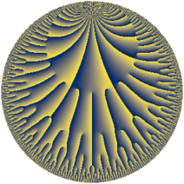# Properties

 Label 21.30.eLevel $21$ Weight $30$ Character orbit 21.e Rep. character $\chi_{21}(4,\cdot)$ Character field $\Q(\zeta_{3})$ Dimension $78$ Newform subspaces $2$ Sturm bound $80$ Trace bound $1$

# Related objects

## Defining parameters

 Level: $$N$$ $$=$$ $$21 = 3 \cdot 7$$ Weight: $$k$$ $$=$$ $$30$$ Character orbit: $$[\chi]$$ $$=$$ 21.e (of order $$3$$ and degree $$2$$) Character conductor: $$\operatorname{cond}(\chi)$$ $$=$$ $$7$$ Character field: $$\Q(\zeta_{3})$$ Newform subspaces: $$2$$ Sturm bound: $$80$$ Trace bound: $$1$$

## Dimensions

The following table gives the dimensions of various subspaces of $$M_{30}(21, [\chi])$$.

Total New Old
Modular forms 158 78 80
Cusp forms 150 78 72
Eisenstein series 8 0 8

## Trace form

 $$78q + 17282q^{2} - 4782969q^{3} - 11434038378q^{4} + 12113760982q^{5} + 313456656384q^{6} - 1515385457433q^{7} - 3851100092424q^{8} - 892194905743479q^{9} + O(q^{10})$$ $$78q + 17282q^{2} - 4782969q^{3} - 11434038378q^{4} + 12113760982q^{5} + 313456656384q^{6} - 1515385457433q^{7} - 3851100092424q^{8} - 892194905743479q^{9} - 394212661060182q^{10} - 2186118177213998q^{11} - 2567836929097728q^{12} + 57935233489473678q^{13} - 25965073904393714q^{14} - 156086879799946644q^{15} - 3178336649346199194q^{16} + 144240220207921992q^{17} + 395356727206636002q^{18} - 14107368967207959825q^{19} + 13921926692753319848q^{20} + 3908937257962756956q^{21} + 173330343337235534196q^{22} + 227411338721107084368q^{23} - 248307981717864522762q^{24} - 1238624059511669383485q^{25} + 1014521889948099025142q^{26} + 218837978263024718418q^{27} + 1283506092388894784082q^{28} + 3323930509411629310408q^{29} + 279585556656013888344q^{30} - 12100635375102358392501q^{31} + 5645376068791944873736q^{32} - 19717706216898333016470q^{33} + 9372546419828643066120q^{34} - 59059452205702458719074q^{35} + 523148245791129820986516q^{36} + 56862380162866449244227q^{37} - 93737882759499371622730q^{38} + 6127634929107980159811q^{39} - 321863004442765222708278q^{40} - 495100379643075046849084q^{41} + 304275995998349057183376q^{42} - 1740812730257647529779734q^{43} - 1133785329805470799832968q^{44} + 277123995834218554131702q^{45} - 2126381570412098109539028q^{46} + 5752666316038044300354558q^{47} + 3345794164179393086102256q^{48} + 11595708112905712593863493q^{49} + 1974845202538182266769748q^{50} - 290020124086466201963280q^{51} + 16719517440592101598523028q^{52} - 3253110181476323422950588q^{53} - 3585441435861396986560512q^{54} + 78863788504745303627376264q^{55} + 66764748927839553827764560q^{56} + 3472272033601410200189574q^{57} + 83109169715120745932399562q^{58} + 7263231905012529002385804q^{59} + 47376743552723710648921734q^{60} - 195520994773149902691190830q^{61} + 1729778225487376551701828580q^{62} - 33192919131296128722162387q^{63} + 2407485555069719044493341380q^{64} + 757132772867700953792231470q^{65} - 11582694508968900447214860q^{66} - 451949283093222270631612131q^{67} - 1039126948605409233555310668q^{68} + 1316325054359907577355054832q^{69} + 3547249376570663577252708330q^{70} - 2181110843915602930916196156q^{71} + 44050408768832486478657732q^{72} + 3353025955820193955102011273q^{73} - 6817124897840710348234001622q^{74} - 2268555367774556053267253847q^{75} + 39218350863665247657962771112q^{76} + 6103646625256030764707307776q^{77} - 4464193239439462421620635708q^{78} + 8803917814556312048973376005q^{79} - 3478925708508696332696936884q^{80} - 20410557688067060951326949319q^{81} - 30856064562964001311763579988q^{82} + 33980785524860350027873021740q^{83} - 24053178283766577802475199720q^{84} + 9180601802105726365318851408q^{85} + 12844278500813508263399299774q^{86} - 6603246166632843316702012740q^{87} - 84052603345211808663404982042q^{88} - 38036605543319829853786081424q^{89} + 18036642460383339169490925804q^{90} - 24231055966985252779215484269q^{91} - 216591026071686206032755183528q^{92} - 10720581159692858646235479615q^{93} - 59471243063537452262469368508q^{94} - 216253630364947624117611021878q^{95} - 57814212822854023790375985846q^{96} - 155196501071485977709285956204q^{97} - 53668444209530951619936442396q^{98} + 100022743644284567515747488156q^{99} + O(q^{100})$$

## Decomposition of $$S_{30}^{\mathrm{new}}(21, [\chi])$$ into newform subspaces

Label Dim. $$A$$ Field CM Traces $q$-expansion
$$a_2$$ $$a_3$$ $$a_5$$ $$a_7$$
21.30.e.a $$38$$ $$111.884$$ None $$25025$$ $$90876411$$ $$-2101591778$$ $$-1\!\cdots\!99$$
21.30.e.b $$40$$ $$111.884$$ None $$-7743$$ $$-95659380$$ $$14215352760$$ $$-368001036334$$

## Decomposition of $$S_{30}^{\mathrm{old}}(21, [\chi])$$ into lower level spaces

$$S_{30}^{\mathrm{old}}(21, [\chi]) \cong$$ $$S_{30}^{\mathrm{new}}(7, [\chi])$$$$^{\oplus 2}$$